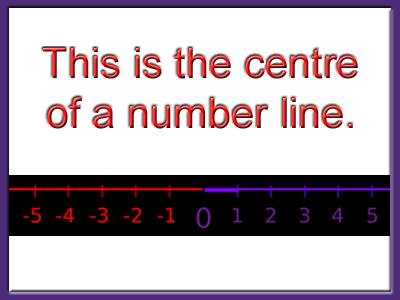Negative numbers are those to the left of zero on a numberline.

# Negative Numbers 3 (Medium)

So, you have made it to the third in our series on Medium Eleven Plus maths quizzes on Negative Numbers – well done! In the previous ones we have only looked at addition and subtraction. In this one we introduce multiplication and division with negative numbers. That may sound daunting, but they are quite simple once you understand the rules!

Here are some tips which will help:

• A negative times a negative equals a positive – e.g. -2 x -2 = 4
• A negative times a positive equals a negative – e.g. -2 x 2 = -4
• A negative divided by a negative equals a positive – e.g. -8 ÷ -2 = 4
• A negative divided by a positive equals a negative – e.g. -8 ÷ 2 = -4

I hope that helps to clarify matters! You would do well to learn these facts – you never know when they might come in useful.

So, now you are armed with the necessary information, it’s time to begin the quiz. Good luck!

1.
What is the correct answer to the given calculation?
17 - 23 + -5 + 10 = ?
-1
0
1
-35
You could group the positive and negative numbers together:
17 - 23 + -5 + 10 = (-23 + -5) + (17 + 10) = -28 = 27 = -1
2.
What is the correct answer to the given calculation?
-5 x -5 = ?
-25
-5
0
25
A negative times a negative equals a positive, so -5 x -5 is the same as 5 x 5
3.
What is the correct answer to the given calculation?
-9 + 13 - -4 = ?
0
4
8
-4
-9 + 13 is the same as 13 - 9, which equals 4. Next, 4 - -4 is the same as 4 + 4, which equals 8
4.
Jon’s bank balance is minus £25 (he owes the bank £25). If he puts £30 into his account, what will Jon’s new balance be?
-£5
-£55
£5
£55
-25 + 30 = 5. Overdrawn bank accounts are one of the places you will come across negative numbers in real life. They are best avoided!
5.
What is the correct answer to the given calculation?
-100 ÷ -10 = ?
10
-10
1,000
-1,000
A negative divided by a negative equals a positive, so -100 ÷ -10 is the same as 100 ÷ 10
6.
What is the correct answer to the given calculation?
-55 - 20 = ?
-75
-35
35
75
55 + 20 = 75, so -55 - 20 = -75
7.
What is the correct answer to the given calculation?
6 x -2 = ?
12
-12
4
-4
6 x 2 = 12, so 6 x -2 = -12
8.
What is the correct answer to the given calculation?
-16 ÷ 4 = ?
4
-4
64
-64
16 ÷ 4 = 4, so -16 ÷ 4 will equal -4
9.
What is the correct answer to the given calculation?
(-4 x 4) ÷ -2 = ?
32
-32
8
-8
(-4 x 4) ÷ -2 = -16 ÷ -2. A negative divided by a negative equals a positive, so -16 ÷ -2 is the same as 16 ÷ 2 = 8
10.
What is the correct answer to the given calculation?
12 - 24 + 8 - -4 = ?
-8
-4
4
0
If we group the positives and negatives then the problem looks like this:
(-24 - -4) + (12 + 8) = -20 + 20 = 0. Remember: subtracting a minus number is the same as adding a positive one
Author:  Frank Evans